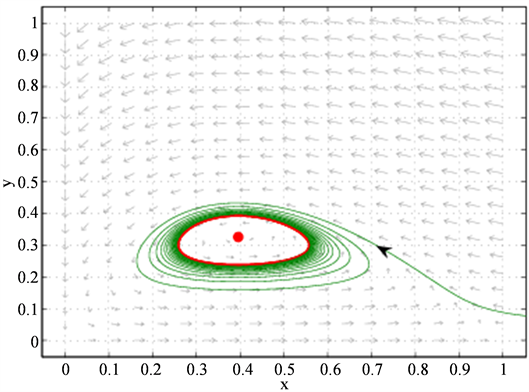# 一类捕食系统中的Hopf分岔Hopf Bifurcation in a Class of Predator-Prey Systems

DOI: 10.12677/AAM.2018.76081, PDF, HTML, XML, 下载: 559  浏览: 754  国家自然科学基金支持

Abstract: In this paper, we studied a class of predator-prey system with a defense mechanism of the prey. By calculating the Lyapunov coefficient, the internal equilibrium point is proved to be a first order weak focus, and the parameter conditions of Hopf bifurcation are given.

1. 引言

Ajraldi  研究了如下的食饵具有牧群行为的广义Holling-II功能函数反应

$\begin{array}{l}\frac{\text{d}x}{\text{d}t}=rx\left(1-\frac{x}{n}\right)-\frac{\alpha \sqrt{x}y}{1+{t}_{h}\alpha \sqrt{x}},\\ \frac{\text{d}y}{\text{d}t}=-sy+\frac{c\alpha \sqrt{x}y}{1+{t}_{h}\alpha \sqrt{x}}.\end{array}$

Braza  研究了简化的食饵含有二次根号防御机制的函数反应

$\begin{array}{l}\frac{\text{d}x}{\text{d}t}=x\left(1-x\right)-\sqrt{x}y,\\ \frac{\text{d}y}{\text{d}t}=-sy+c\sqrt{x}y,\end{array}$

$\begin{array}{l}\frac{\text{d}x}{\text{d}t}=x\left(1-x\right)-\sqrt{x}y,\\ \frac{\text{d}y}{\text{d}t}=-sy+c\sqrt{x}y.\end{array}$ (1)

2. 主要结果

$J\left(x,y\right)=\left[\begin{array}{cc}1-2x-\frac{1}{3}{x}^{-\frac{2}{3}}y& -{x}^{\frac{1}{3}}\\ \frac{1}{3}c{x}^{-\frac{2}{3}}y& -s+c{x}^{\frac{1}{3}}\end{array}\right].$ (2)

Hopf分岔出的稳定的极限环。

$J\left(E\left({x}^{*},{y}^{*}\right)\right)=\left[\begin{array}{cc}\frac{2{c}^{3}-5{s}^{3}}{3{c}^{3}}& -\frac{s}{c}\\ \frac{{c}^{3}-{s}^{3}}{3{c}^{2}}& 0\end{array}\right].$ (3)

$\sigma \left(s\right)=trJ\left(E\left({x}^{*},{y}^{*}\right)\right),\Delta \left(s\right)=\mathrm{det}J\left(E\left({x}^{*},{y}^{*}\right)\right),\mu \left(s\right)=\frac{1}{2}\sigma \left(s\right),\omega \left(s\right)=\frac{1}{2}\sqrt{4\Delta \left(s\right)-{\sigma }^{2}\left(s\right)}$

$\mu \left(s\right)=\frac{1}{2}\sigma \left(s\right)=\frac{2{c}^{3}-5{s}^{3}}{6{c}^{3}},$

${s}_{0}={\left(\frac{2}{5}\right)}^{\frac{1}{3}}c$ 时， $\mu \left({s}_{0}\right)=0$ ，可得

$\omega \left({s}_{0}\right)=\sqrt{\frac{c}{5}×{\left(\frac{2}{5}\right)}^{\frac{1}{3}}}>0.$

${\mu }^{\prime }\left({s}_{0}\right)=-\frac{5}{2c}×{\left(\frac{2}{5}\right)}^{\frac{2}{3}}<0.$

$s={s}_{0}$ 时，平衡点 $E\left({x}^{*},{y}^{*}\right)$ 的坐标为

$E\left({x}^{*},{y}^{*}\right)=\left(\frac{2}{5},\frac{3}{5}×{\left(\frac{2}{5}\right)}^{\frac{2}{3}}\right).$

$\begin{array}{l}x=\frac{2}{5}+{\xi }_{1},\\ y=\frac{3}{5}×{\left(\frac{2}{5}\right)}^{\frac{2}{3}}+{\xi }_{2}.\end{array}$

$\begin{array}{l}{{\xi }^{\prime }}_{1}=-{\left(\frac{2}{5}\right)}^{\frac{1}{3}}{\xi }_{2}-\frac{5}{6}{\xi }_{1}{}^{2}-\frac{1}{3}×{\left(\frac{2}{5}\right)}^{-\frac{2}{3}}{\xi }_{1}{\xi }_{2}-\frac{25}{108}{\xi }_{1}{}^{3}+\frac{1}{9}{\left(\frac{2}{5}\right)}^{-\frac{5}{3}}{\xi }_{1}{}^{2}{\xi }_{2}+o\left({|{\xi }_{1},{\xi }_{2}|}^{4}\right),\\ {{\xi }^{\prime }}_{2}=\frac{c}{5}{\xi }_{1}-\frac{c}{6}{\xi }_{1}{}^{2}+\frac{c}{3}×{\left(\frac{2}{5}\right)}^{-\frac{2}{3}}{\xi }_{1}{\xi }_{2}+\frac{25c}{108}{\xi }_{1}{}^{3}-\frac{c}{9}×{\left(\frac{2}{5}\right)}^{-\frac{5}{3}}{\xi }_{1}{}^{2}{\xi }_{2}+o\left({|{\xi }_{1},{\xi }_{2}|}^{4}\right).\end{array}$ (4)

${\xi }^{\prime }=A\xi +\frac{1}{2}B\left(\xi ,\xi \right)+\frac{1}{6}C\left(\xi ,\xi ,\xi \right),$

$B\left(\xi ,\eta \right)=\left(\begin{array}{c}-\frac{5}{3}{\xi }_{1}{\eta }_{1}-\frac{1}{3}{\left(\frac{2}{5}\right)}^{-\frac{2}{3}}\left({\xi }_{1}{\eta }_{2}+{\xi }_{2}{\eta }_{1}\right)\\ -\frac{c}{3}{\xi }_{1}{\eta }_{1}+\frac{c}{3}{\left(\frac{2}{5}\right)}^{-\frac{2}{3}}\left({\xi }_{1}{\eta }_{2}+{\xi }_{2}{\eta }_{1}\right)\end{array}\right),$ (5)

$C\left(\xi ,\eta ,\delta \right)=\left(\begin{array}{c}-\frac{25}{16}{\xi }_{1}{\eta }_{1}{\delta }_{1}+\frac{2}{9}{\left(\frac{2}{5}\right)}^{-\frac{5}{3}}\left({\xi }_{1}{\eta }_{1}{\delta }_{2}+{\xi }_{1}{\eta }_{2}{\delta }_{1}+{\xi }_{2}{\eta }_{1}{\delta }_{1}\right)\\ -\frac{25}{16}{\xi }_{1}{\eta }_{1}{\delta }_{1}-\frac{2c}{9}{\left(\frac{2}{5}\right)}^{-\frac{5}{3}}\left({\xi }_{1}{\eta }_{1}{\delta }_{2}+{\xi }_{1}{\eta }_{2}{\delta }_{1}+{\xi }_{2}{\eta }_{1}{\delta }_{1}\right)\end{array}\right)$ (6)

$A=\left(\begin{array}{cc}0& -{\left(\frac{2}{5}\right)}^{\frac{1}{3}}\\ \frac{c}{5}& 0\end{array}\right)=\left(\begin{array}{cc}0& -{\left(\frac{2}{5}\right)}^{\frac{1}{3}}\\ {\omega }^{2}{\left(\frac{5}{2}\right)}^{\frac{1}{3}}& 0\end{array}\right),$ (7)

$q=\left(\begin{array}{c}{2}^{\frac{1}{3}}\\ -i\omega {5}^{\frac{1}{3}}\end{array}\right),\text{\hspace{0.17em}}p=\frac{1}{2\omega {5}^{\frac{1}{3}}{2}^{\frac{1}{3}}}\left(\begin{array}{c}{5}^{\frac{1}{3}}\omega \\ -i{2}^{\frac{1}{3}}\end{array}\right),$ (8)

$〈p,q〉=1$ 。由(5)，(6)，(8)可得

$B\left(q,q\right)=\left(\begin{array}{c}-\frac{5}{3}×{2}^{\frac{2}{3}}+\frac{5i\omega }{3}×{2}^{\frac{2}{3}}\\ -\frac{c}{3}×{2}^{\frac{2}{3}}-\frac{5ci\omega }{3}×{2}^{\frac{2}{3}}\end{array}\right),B\left(q,\stackrel{¯}{q}\right)=\left(\begin{array}{c}-\frac{5}{3}×{2}^{\frac{2}{3}}\\ -\frac{c}{3}×{2}^{\frac{2}{3}}\end{array}\right),$ (9)

$C\left(q,q,\stackrel{¯}{q}\right)=\left(\begin{array}{c}-\frac{25}{9}-\frac{25i\omega }{9}\\ -\frac{25c}{9}+\frac{25ciw}{9}\end{array}\right).$ (10)

$\begin{array}{l}{g}_{20}=〈p,B\left(q,q\right)〉=-\frac{5}{3}×{2}^{-\frac{2}{3}}+\frac{5i\omega }{3}-\frac{\frac{ic}{3}×{2}^{-\frac{1}{3}}}{{5}^{\frac{1}{3}}\omega }+\frac{{5}^{\frac{2}{3}}×{2}^{\frac{1}{3}}c}{3},\\ {g}_{11}=-\frac{5}{3}×{2}^{-\frac{2}{3}}-\frac{\frac{ic}{3}×{2}^{-\frac{1}{3}}}{{5}^{\frac{1}{3}}\omega },{g}_{21}=-\frac{27}{16}-\frac{27}{8}i\omega +\frac{9\sqrt{3}ci}{16\omega }-\frac{9\sqrt{3}c}{8}.\end{array}$Figure 1. Stable limit cycle phase diagram of bifurcation from equilibrium point, bifurcation parameter is $s=0.734,c=1$

${l}_{1}\left({s}_{0}\right)=\frac{1}{2{\omega }^{2}}\mathrm{Re}\left(i{g}_{20}{g}_{11}+\omega {g}_{21}\right)=-\frac{{5}^{\frac{2}{3}}c}{18{\omega }^{3}}<0,$

$E\left({s}_{0}\right)$ 为一阶细焦点，所以，当 $s<{s}_{0}$ 时，从平衡点 $E\left({x}^{*},{y}^{*}\right)$ 分岔出一个稳定的极限环，参看图1

  Ajraldi, V., Pittavino, M. and Venturino, E. (2011) Modeling Herd Behavior in Population Systems. Nonlinear Analysis: Real World Applications, 12, 2319-2338. https://doi.org/10.1016/j.nonrwa.2011.02.002  Braza, P.A. (2012) Predator-Prey Dynamics with Square Root Functional Responses. Nonlinear Analysis: Real World Applications, 13, 1837-1843. https://doi.org/10.1016/j.nonrwa.2011.12.014  Kuznetsov, Y.A. (2004) Elements of Applied Bifurcation Theory. Spring-er-Verlag, New York. https://doi.org/10.1007/978-1-4757-3978-7  张芷芬, 丁同仁, 黄文灶, 董镇喜, 微分方程定性理论[M]. 北京: 科学出版社, 1981.  张伟年, 杜正东, 徐冰, 常微分方程[M]. 北京: 高等教育出版社, 2006.